Processing ......FreeComputerBooks.com Free Computer, Mathematics, Technical Books and Lecture Notes, etc.

Applied MathematicsRelated Book Categories:
•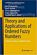Theory and Applications of Ordered Fuzzy Numbers

This book offers comprehensive coverage on Ordered Fuzzy Numbers, providing readers with both the basic information and the necessary expertise to use them in a variety of real-world applications.

•Street-Fighting Math: Guessing & Opportunistic Problem Solving

This engaging book is an antidote to the rigor mortis brought on by too much mathematical rigor, teaching us how to guess answers without needing a proof or an exact calculation.

•Statistical Methodologies and Their Application to Real Problems

This book provides a cross-disciplinary forum for exploring the variety of new data analysis techniques emerging from different fields, focusing on recent advances in data analysis techniques in many different research fields.

•Fourier Transforms - High-tech Application and Current Trends

The main purpose of this book is to provide a modern review about recent advances in Fourier transforms as the most powerful analytical tool for high-tech application in electrical, electronic, and computer engineering, as well as other applications.

•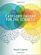Category Theory for the Sciences (David I. Spivak)

Using databases as an entry to category theory, this book explains category theory by examples, and shows that category theory can be useful outside of mathematics as a rigorous, flexible, and coherent modeling language throughout the sciences.

•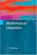Mathematical Linguistics (Andras Kornai)

This book introduces the mathematical foundations of linguistics to computer scientists, engineers, and mathematicians interested in natural language processing (NLP). It presents linguistics as a cumulative body of knowledge from the ground up.

•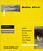Math Alive (Ingrid Daubechies, et at)

Mathematics has profoundly changed our world, from banking to listening to music. This course is designed for those who haven't had college mathematics but would like to understand some of the mathematical concepts behind important modern applications.

•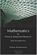Mathematics for Classical Information Retrieval: Roots and Apps

This book is about Information Retrieval (IR), particularly Classical Information Retrieval (CIR). The primary goal of book is to create a context for understanding the principles of CIR by discussing its mathematical bases.

•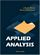Applied Analysis (John K. Hunter, et al)

This book provides an introduction to those parts of analysis that are most useful in applications for graduate students. The material is selected for use in applied problems, and is presented clearly and simply but without sacrificing mathematical rigor.

•Applied Linear Algebra in Action (Vasilios N. Katsikis)

This textbook contains a collection of six high-quality chapters. The techniques are illustrated by a wide sample of applications. It is devoted to Linear Mathematics by presenting problems in Applied Linear Algebra of general or special interest.

•Applied Linear Algebra: Vectors, Matrices, and Least Squares

Combines straightforward explanations with a wealth of practical examples to offer an innovative approach to teaching linear algebra. Requiring no prior knowledge of the subject, it covers the aspects of linear algebra - vectors, matrices, and least squares

•Linear Algebra: A Course for Physicists and Engineers (Arak Mathai)

This textbook on linear algebra is written to be easy to digest by non-mathematicians. It introduces the concepts of vector spaces and mappings between them without too much theorems and proofs. Various applications of the formal theory are discussed as well.

•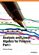Analysis and Linear Algebra for Finance (Patrick Roger)

This book presents the elements of analysis and linear algebra used in financial models and in microeconomics. Functions of one and several variables and matrices are developed as well as vector spaces, linear mappings and optimization methods, etc.

•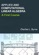Applied and Computational Linear Algebra: A First Course

This book is intended as a text for a graduate course that focuses on applications of linear algebra and on the algorithms used to solve the problems that arise in those applications.

•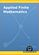Applied Finite Mathematics (Rupinder Sekhon)

This book covers topics including linear equations, matrices, linear programming (geometrical approach and simplex method), the mathematics of finance, sets and counting, probability, Markov chains, and game theory.

•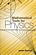Mathematical Tools for Physics (James Nearing)

This book helps physics students learn to take an informed and intuitive approach to solving problems. It assists undergraduates in developing their skills and provides them with grounding in important mathematical methods.

•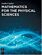Mathematics for the Physical Sciences (Leslie Copley)

This book provides a comprehensive introduction to the areas of mathematical physics. It combines all the essential math concepts into clearly written reference and illustrates the mathematics with numerous physical examples drawn from contemporary research.

•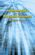Mathematics for the Physical Sciences (Herbert S. Wilf)

This book provides a text for a first-year graduate level course in mathematical methods. Advanced undergraduates and graduate students in the natural sciences will receive a solid foundation in several fields of mathematics with this text.

•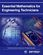Essential Mathematics for Engineers (W. J. R. H. Pooler)

This book explains mathematical jargon and develops the formulae used by engineers from first principles. The first chapter is a summary so the reader can quickly see where further study is needed. The book is in two parts, pure and applied.

•Essential Engineering Mathematics (Michael Batty)

The aim is more to highlight and explain some areas commonly found difficult, such as calculus, and to ease the transition from school level to university level mathematics, where sometimes the subject matter is similar, but the emphasis is usually different.

•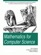Mathematics for Computer Science (Eric Lehman, et al)

This book covers elementary discrete mathematics for computer science and engineering. It emphasizes mathematical definitions and proofs as well as applicable methods. explores the topics of basic combinatorics, number and graph theory, logic and proof techniques.

•Shape Interrogation for Computer Aided Design and Manufacturing

This book provides the mathematical fundamentals as well as algorithms for various shape interrogation methods including nonlinear polynomial solvers, intersection problems, differential geometry of intersection curves, distance functions etc.

•Strange Attractors: Creating Patterns in Chaos (Julien C. Sprott)

This book describes a simple method for generating an endless succession of beautiful fractal patterns by iterating simple maps and ordinary differential equations with coefficients chosen automatically by the computer.

•Mathematics in the Age of the Turing Machine (Thomas C. Hales)

Computers have rapidly become so pervasive in mathematics that future generations may look back to this day as a golden dawn. The article gives a survey of mathematical proofs that rely on computer calculations and formal proofs.

•Data Assimilation: A Mathematical Introduction (Kody Law, et al)

This book provides a systematic treatment of the mathematical underpinnings of work in data assimilation, covering both theoretical and computational approaches. Specifically the authors develop a unified mathematical framework of Bayesian formulation.

•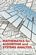Mathematics for Algorithm and Systems Analysis (E. A. Bender)

This up-to-date textbook assists undergraduates in mastering the ideas and mathematical language to address problems that arise in the field's many applications.

•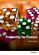Probability for Finance (Patrick Roger)

This book provides technical support for students in finance. It reviews the main probabilistic tools used in financial models in a pedagogical way, starting from simple concepts like random variables and tribes and going to more sophisticated ones like conditional expectations and limit theorems.

•Stochastic Processes for Finance (Patrick Roger)

It describes the most important stochastic processes used in finance in a pedagogical way, especially Markov chains, Brownian motion and martingales. It also shows how mathematical tools like filtrations, Ito's lemma or Girsanov theorem should be understood in the framework of financial models.

•Mathematical Applications for Game Development (R. Baldwin)

This book presents applications of mathematics and science in game and simulation programming. Includes the utilization of matrix and vector operations, kinematics, and Newtonian principles in games and simulations. Also covers code optimization.

•Vector Math for 3D Computer Graphics, 4th Revision (Bradley Kjell )

This is a tutorial on vector algebra and matrix algebra from the viewpoint of computer graphics. It covers most vector and matrix topics needed to read college-level computer graphics text books.

•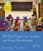3D Math Primer for Graphics and Game Development (F. Dunn)

Working C++ classes for mathematical and geometric entities and several different matrix classes illustrate how to put the techniques into practice, and exercises at the end of each chapter help reinforce the 3D programming concepts.

•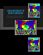Linear Mathematics In Infinite Dimensions (Ulrich H. Gerlach)

Focus on the mathematical framework that underlies linear systems arising in physics, engineering and applied mathematics - from the theory of linear transformation on finite dimensional vector space to the infinite dimensional vector spaces.

•Numerical Simulations of Physical and Engineering Processes

The book handles the numerical simulations of physical and engineering systems. It can be treated as a bridge linking various numerical approaches of two closely inter-related branches of science, i.e. physics and engineering.

•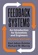Feedback Systems: An Introduction for Scientists and Engineers

This book provides an introduction to the mathematics needed to model, analyze, and design feedback systems. It uses techniques from physics, computer science, and operations research to introduce control-oriented modeling.

•Games, Fixed Points and Mathematical Economics (C. Ewald)

This book gives the reader access to the mathematical techniques involved and goes on to apply fixed point theorems to proving the existence of equilibria for economics and for co-operative and noncooperative games.

•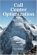Call Center Mathematics: Understanding and Improving

This book gives an overview of the role and potential of mathematical optimization in call centers. It deals extensively with all aspects of workforce management, but also with topics such as call routing and the scheduling of multiple channels.

•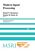Modern Signal Processing (Daniel N. Rockmore, et al)

Signal processing is ubiquitous in modern technology. Its mathematical basis and applications are the subject of this book. Emphasis is on challenges, new techniques adapted to new technologies, and certainrecent advances in algorithms and theory.

•One Two Three ... Infinity: Facts and Speculations of Science

Whatever your level of scientific expertise, chances are you'll derive a great deal of pleasure, stimulation, and information from this unusual and imaginative book. It belongs in the library of anyone curious about the wonders of the scientific universe.

•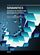Semantics - Advances in Theories and Mathematical Models

This book is a nice blend of number of great ideas, theories, mathematical models, and practical systems in the domain of Semantics, which highlights the advances in theories and mathematical models in the domain of Semantics.

•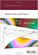Mathematics and Music (David Wright)

An investigation of the interrelationships between mathematics and music, reviewing the needed background concepts in each subject as they are encountered. It explores the common foundations of the two subjects, which are developed side by side.

•Music: A Mathematical Offering (David J. Benson)

This book provides a wealth of information to understand, at varying levels of technicality, the interplay between two ancient disciplines. A must-have book if you want to know about the music of the spheres or digital music and much in between.

•How Music and Mathematics Relate (David Kung)

Understanding the connections between music and mathematics helps you appreciate both, even if you have no special ability in either field - from knowing the mathematics behind tuning an instrument to understanding the features that define your favorite pieces.

•Modeling with Data: Tools and Techniques for Scientific Computing

Modeling with Data fully explains how to execute computationally intensive analyses on very large data sets, showing readers how to determine the best methods for solving a variety of different problems, etc. with powerful statistical and mathematical tools.

•Applied Mathematics

This is the previous page of Applied Mathematics, we are in the processing to convert all the books there to the new page. Please check this page again!!!

Book CategoriesAll CategoriesRecent BooksIT Research LibraryMiscellaneous Books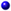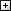Computer LanguagesComputer ScienceData Science/DatabasesElectronic EngineeringJava and Java EE (J2EE)Linux and UnixMathematicsMicrosoft and .NETMobile ComputingNetworking and CommunicationsSoftware EngineeringSpecial TopicsWeb Programming
Other Categories